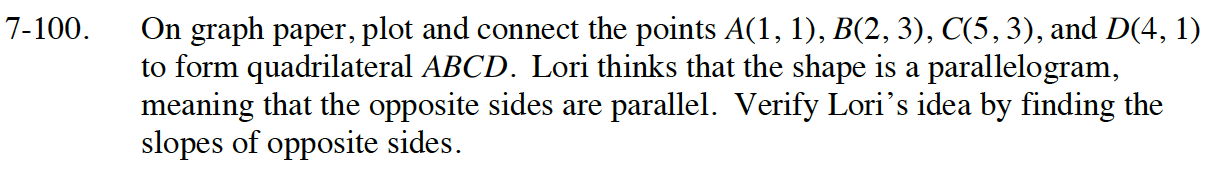Home > CC4 > Chapter 7 > Lesson 7.2.2 > Problem7-100

7-100.

On graph paper, plot and connect the points A(1, 1), B(2, 3), C(5, 3), and D(4, 1) to form quadrilateral ABCD. Lori thinks that the shape is a parallelogram, meaning that the opposite sides are parallel. Verify Lori’s idea by finding the slopes of opposite sides. 7-100 HW eTool (Desmos). Homework Help ✎Read Math Notes box in Lesson 2.1.4 about how to calculate slope.

Use the eTool below to help solve the problem.
Click the link at right for the full version of the eTool: Int1 7-100 HW eTool